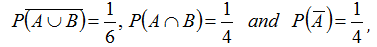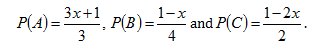Select Page

Introduction:
Probability is a super-fascinating topic that keeps on your toes. Along with mathematical ability, it requires a combination of quick thinking and reasoning skills in order to crack these exams quickly. An important part of CAT quantitative aptitude section, it is important you practice problems for this topic. We bring this five-problem set with the same intent and purpose.
Question 1: Let A and B be two events such thatWhere  Ā stands for complement of event A. Then, events A and B are
(a) Mutually exclusive
(b) Independent but not equally likely
(c) Equally likely but not independent
(d) Equally likely and mutually exclusive

Question 2: Three houses are available in a locality. Three persons apply for the houses. Each applies for one house without consulting others. The probability that all the three apply for the same house, is
(a) 7/9
(b) 8/9
(c) 1/9
(d) 2/9

Option c

All the three persons has three options to apply a house.

Therefore total number of cases = 33

Now, favorable cases = 3 (All can apply for house 1 or 2 or 3)

Therefore required probability = {3/(33)} = 1/9

Question 3: The probability that A speaks truth is 4/5 while this probability for B is 3/4. The probability that they contradict each other when asked to speak on a fact, is
(a) 7/20
(b) 1/5
(c) 3/20
(d) 4/5

Question 4: Five horses are in a race. Mr. A selects two of the horses at random and bets on them. The probability that Mr. A selected the winning horse, is
(a) 4/5
(b) 3/5
(c) 1/5
(d) 2/5

Option (d)

The probability that Mr. A selected the loosing horse = 4/5 x ¾ = 3/5

The probability that Mr. A selected the winning horse = {1 – (3/5)} = 2/5

Question 5: Events A, B, C are mutually exclusive events such thatThe set of possible values of x are in the interval

(a) 1/3 , ½
(b) 1/3, 2/3
(c) 1/3, 13/3
(d) 0,1

Tips and Tricks you can use CAT Probability Problems

• Do remember that probability is not a pure mathematics topics. It requires you to combine reasoning skills with mathematical ability.
• Some important areas that you should cover to solve CAT Probability Problems: dices, playing cards, and more. A number of problems are based on these concepts for this topic.
• The best resource for learning the basics of Probability is class 11/12 books. You can refer to books by RD Sharma and NCERT to understand the basics of the topic.
• One of the best books for probability is by KC Sinha. It adopts a comprehensive approach for the topic and you can refer to it mastering the topic.
• Make sure you study Permutation and Combinations. Probability is based on the basics of P and C and therefore, you need to make sure you study this topic.
Get Posts Like This Sent to your Email
Updates for Free Live sessions and offers are sent on mail. Don't worry: we do not send too many emails..:)
Get Posts Like This Sent to your Email
Updates for Free Live sessions and offers are sent on mail. Don't worry: we do not send too many emails..:)